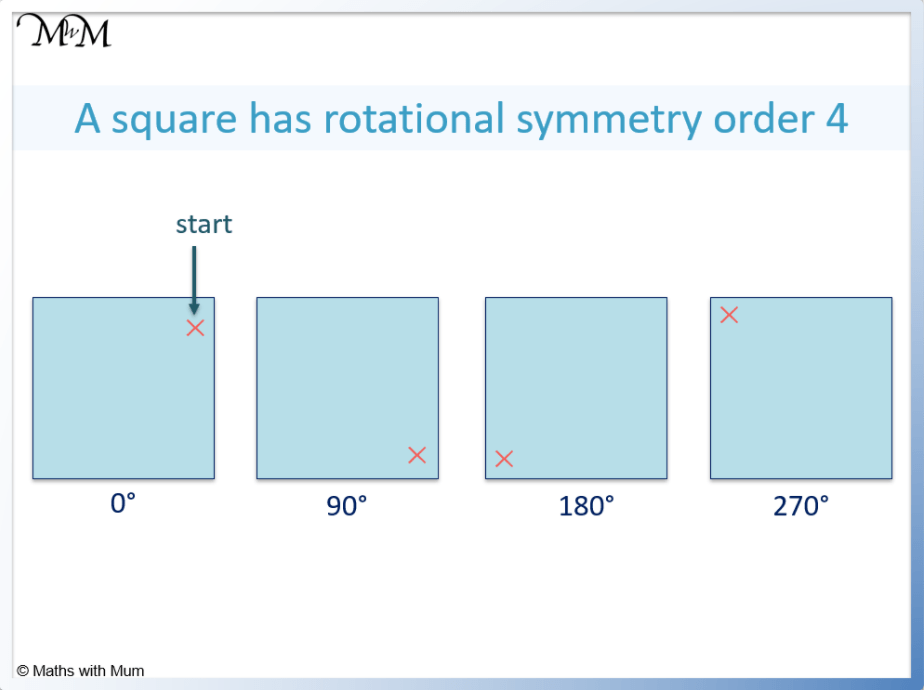# How to Rotate a Shape

How to Rotate a ShapeRotating a shape means to turn it to face a different direction. The size and shape do not change.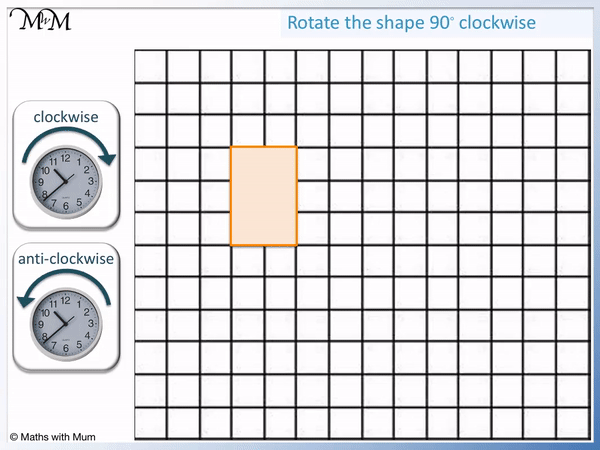• Rotating a shape means to spin it around so that it is facing a different direction.
• The size and the shape of the rotated shape do not change.
• Clockwise rotations turn in the same direction as the hands on a clock.
• Counter-clockwise (or anti-clockwise) rotations turn in the opposite direction to the hands on a clock.
• 90° is one-quarter of a full turn. 180° is half a full turn. 270° is three-quarters of a full turn.• To rotate a shape 90° clockwise, turn it a quarter of a full turn in the same direction as the hands of a clock.
• Cover the shape in tracing paper and draw around it.
• Draw an upwards facing arrow from the centre of rotation.
• When rotating 90° clockwise, this arrow will turn from facing upwards to facing right.
• Draw the shape in its new position once the arrow is facing right.#### Video Lesson: How to Rotate a Shape About a Point# Rotating Shapes

## What Does it Mean to Rotate a Shape?

Rotating a shape means to change its direction by turning it. The size and shape do not change during rotation. A shape is often rotated about a specific point called the centre of rotation. We also need to know what angle and direction the shape is rotated in.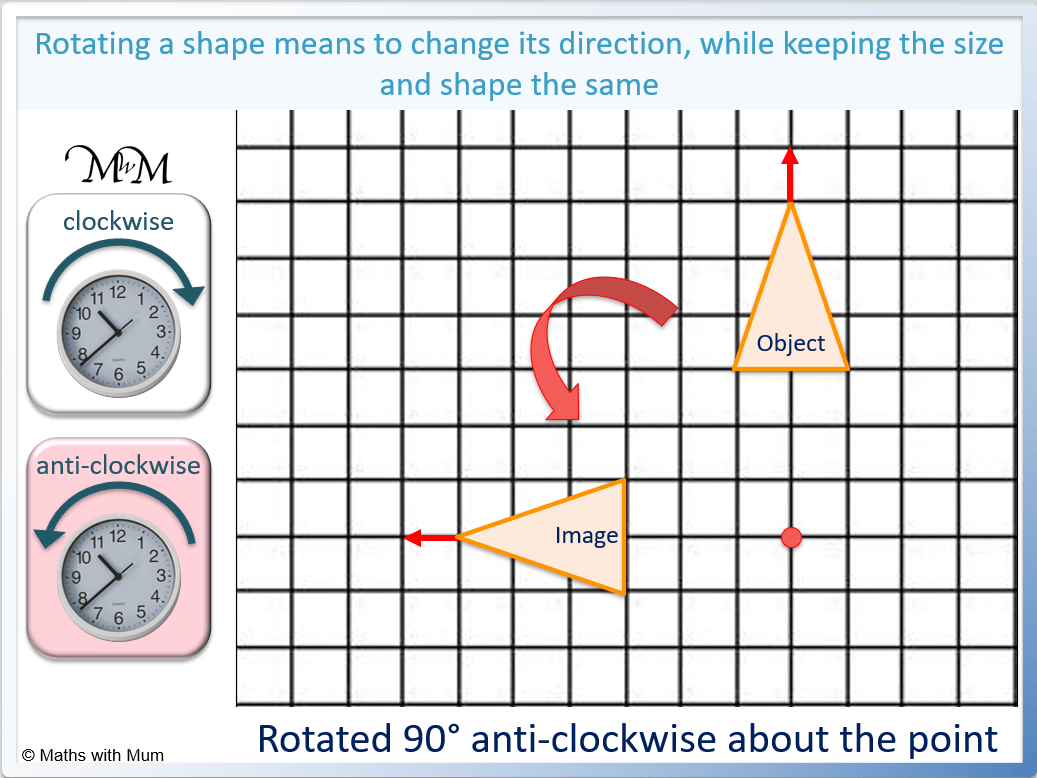For example, this triangle has been rotated 90° counter-clockwise about the point.

You can tell that a shape has been rotated because it is not facing the same direction as it was originally. The original shape is called the object and the rotated shape is called the image.

When rotated, a shape remains the same distance away from the centre of rotation. It is just in a different direction.## Rules for Rotating a Shape About the Origin

The rules for rotating shapes using coordinates are:

Clockwise rotation angle Counter-clockwise rotation angle Rule
90° 270° (x, y) → (y, -x)
180° 180° (x, y) → (-x, -y)
270° 90° (x, y) → (-y, x)

#### How to Rotate a Shape by 90 Degrees

To rotate shape 90° clockwise about the origin, all original coordinates (x, y) becomes (y, -x). To rotate a shape 90° counter-clockwise about the origin, the coordinates (x, y) become (-y, x). Simply switch the x and y coordinates and multiply the coordinate with the negative sign by -1.

For example, use the rule (x, y) to (y, -x) to rotate the shape 90° clockwise.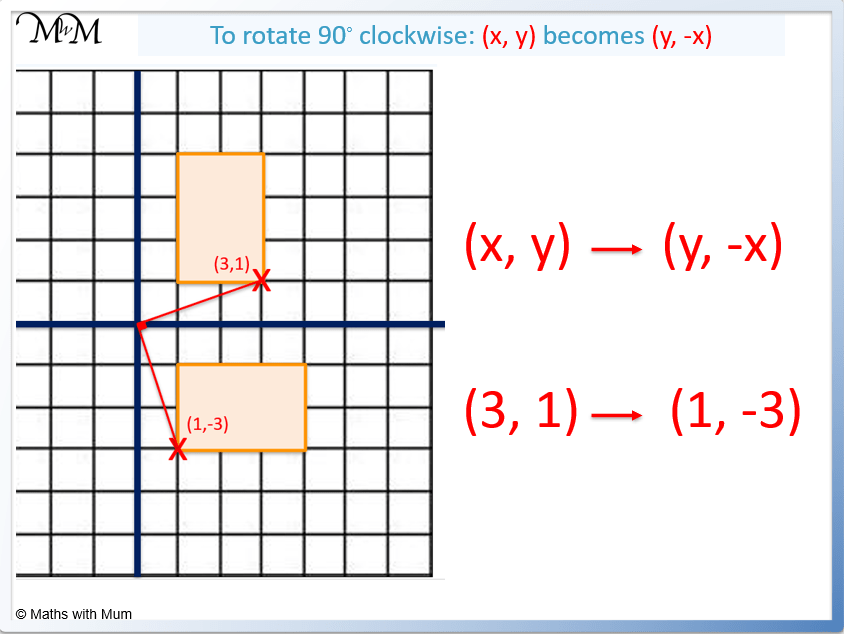To use this rule, simply switch the (3, 1) to (1, 3) and then make the 3 negative to get (1, -3).

#### How to Rotate a Shape by 180 Degrees

To rotate a shape by 180° clockwise or counter-clockwise, the rule is to replace the (x, y) coordinates with (-x, -y). For example, a coordinate at (3, 1) will move to (-3, -1) after a 180° rotation.Simply multiply each coordinate by -1 to rotate a shape 180°.

If a coordinate is negative, it will become positive after a 180° rotation. For example, the coordinate (-1, -4), will move to (1, 4) after a 180° rotation.

#### How to Rotate a Shape by 270 Degrees

To rotate shape 270° clockwise about the origin, all original coordinates (x, y) becomes (-y, x). To rotate a shape 270° counter-clockwise about the origin, the coordinates (x, y) become (y, -x). Simply switch the x and y coordinates and multiply the coordinate with the negative sign by -1.

For example, to rotate the point (3, 1) 270° clockwise, it becomes (-1, 3). Simply switch the x and y coordinates to get (1, 3) and then multiply the 1 by -1 to get (-1, 3).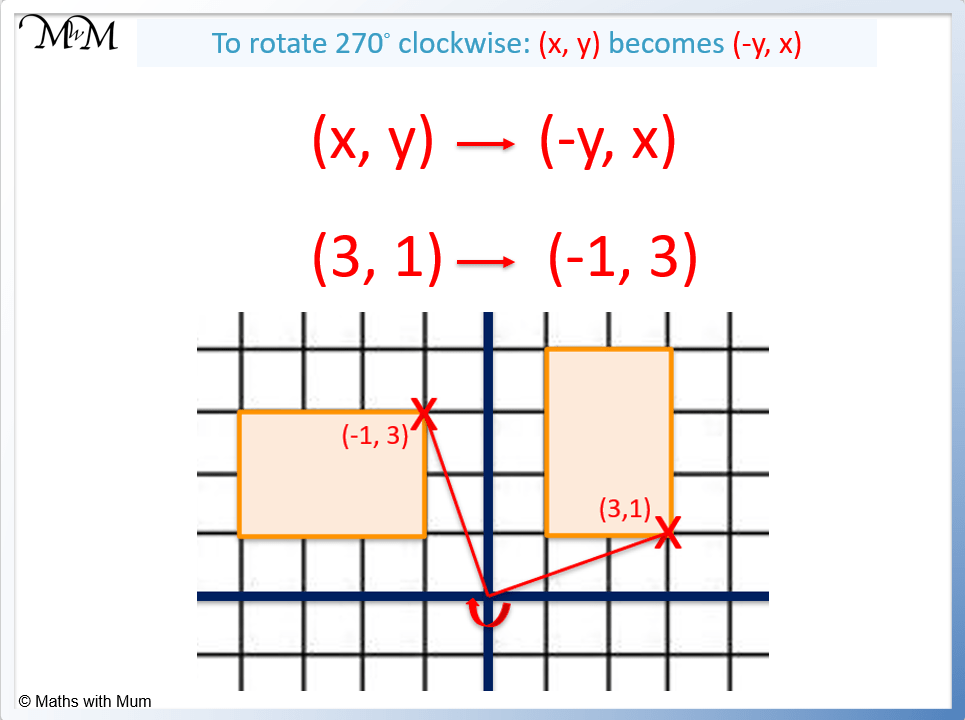## How to Rotate a Shape Using Tracing Paper

To rotate a shape using tracing paper:

1. Place the tracing paper over the shape and draw around the shape.
2. Draw an arrow from the centre of rotation pointing upwards.
3. Keep the pen over the centre of rotation and rotate the tracing paper.
4. Stop when the arrow is facing either right (for 90° CW / 270° CCW turn), down (for 180° turn) or left (for 270° CW / 90° CCW turn).
5. Draw the shape in this new position below the tracing paper.

The easiest way to rotate a shape is to use tracing paper.

For example, use tracing paper to rotate the shape 90° clockwise about the point.After a 90° clockwise rotation, the upwards arrow is facing right. Use the grid lines of the paper to help line up the arrow correctly, ensuring that it is completely horizontal.

Then draw in the shape below.

Here is another example. Rotate the shape 270° clockwise about the point using tracing paper.Every lot of 90° is equivalent to a quarter turn. 270° is 3 lots of 90° and so, 270° is equivalent to three-quarters of a turn.

The upward-facing arrow will be facing to the left after a 270° clockwise rotation.

We draw the shape in below.

## How to Rotate a Shape Without Using Tracing Paper

To rotate a shape without tracing paper, draw horizontal and vertical arrows from the centre of rotation to each corner of the shape. The new corner can be found by rotating each of these arrows according to the following rules:

Original Direction 90° CW / 270° CCW 180° CW / 180 ° CCW 270° CW / 90 ° CCW

For example, rotate the shape 90° clockwise without using tracing paper.

The first step is to draw horizontal and vertical arrows connecting the centre of rotation to a corner on the shape.

The corner selected below is one square right and one square up from the centre of rotation.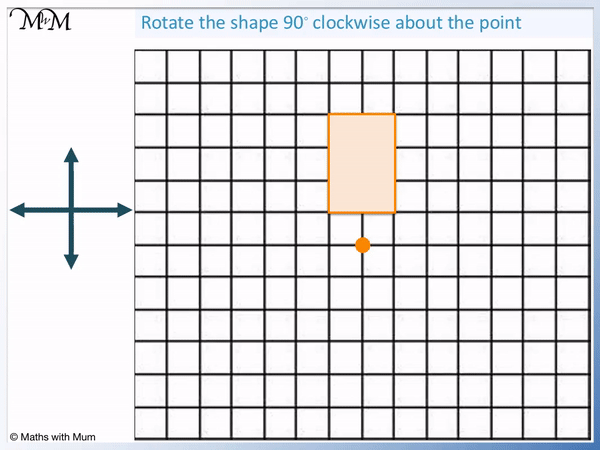Using the rules above, a right-facing arrow will be facing down following a 90° clockwise rotation. An upwards-facing arrow will be facing right after a 90° clockwise rotation.

Instead of the corner being one right and one up, it will now be one down and one right.

Using the new position of this corner, the rest of the shape can be drawn in. The same process can be repeated for all corners to check the result.

Here is another example. Rotate the shape 270° clockwise without using tracing paper.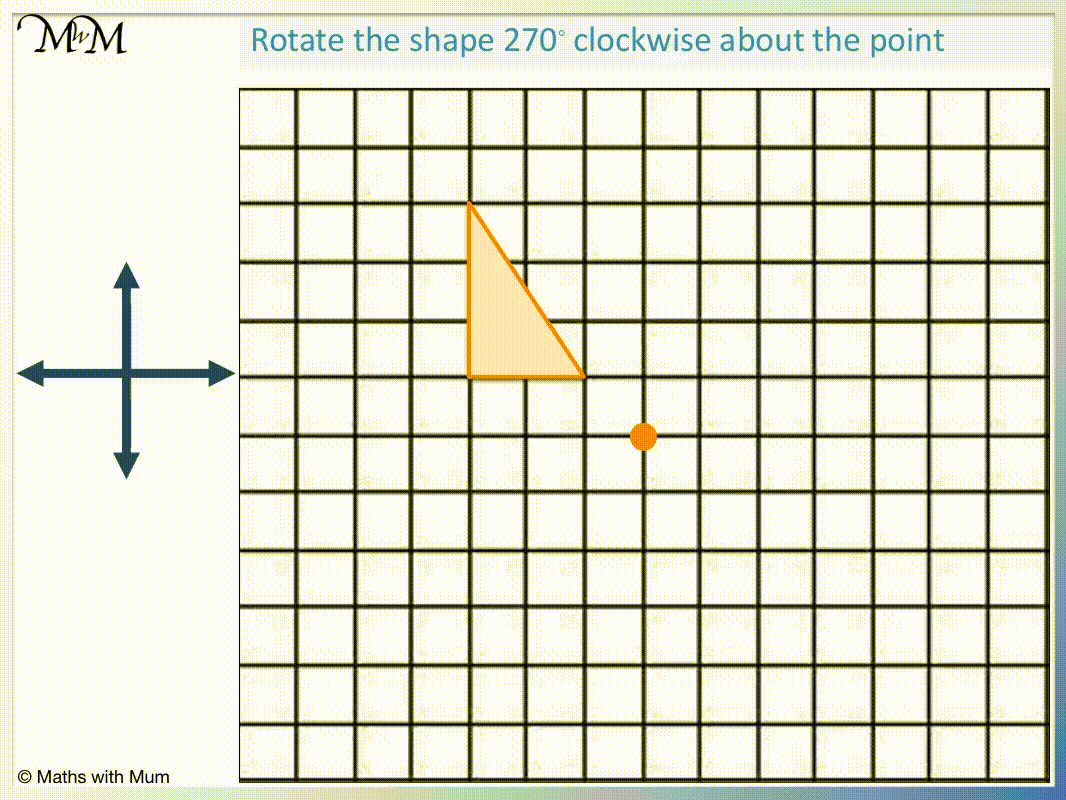Draw horizontal and vertical arrows from the centre of rotation to each corner.

After a 270° clockwise rotation all upwards-facing arrows will be facing left and all left-facing arrows will be facing down.

Once all corners are drawn in their new positions, the rotated shape can be drawn by connecting these together.Now try our lesson on Rotational Symmetry where we learn how to find the order of rotational symmetry for a shape.